Q.1) Determine, the type of Triangle for the given figure ?​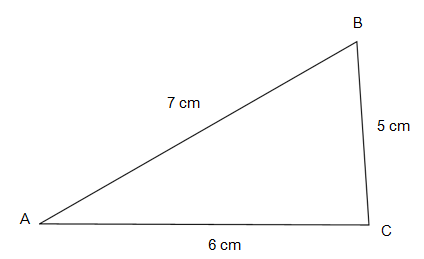a) Equilateral Triangle
b) Isosceles Triangle
c) Scalene Triangle

Q.2) Determine, the type of Triangle for the given figure ?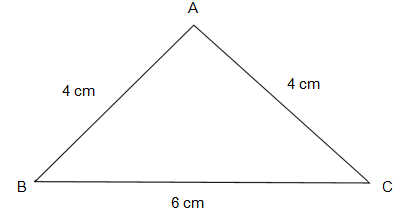a) Equilateral Triangle
b) Isosceles Triangle
c) Scalene Triangle

Q.3) Determine, the type of Triangle for the given figure ?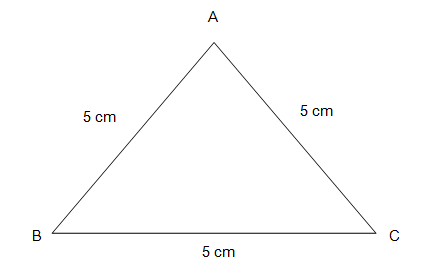a) Equilateral Triangle
b) Isosceles Triangle
c) Scalene Triangle

Q.4) Determine, the type of Triangle for the given figure ?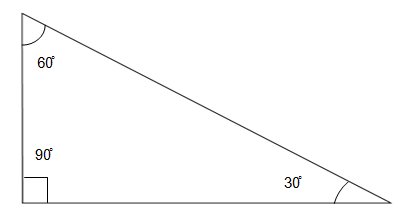a) Acute Angled Triangle
b) Obtuse Angled Triangle
c) Right Angled Triangle

Q.5) Determine, the type of Triangle for the given figure ?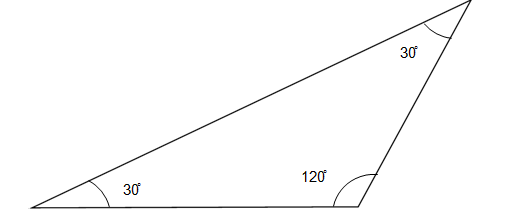a) Obtuse Angled Triangle
b) Acute Angled Triangle
c) Right Angled Triangle

Q.6) Determine whether the given figure is a Square or Rectangle  ?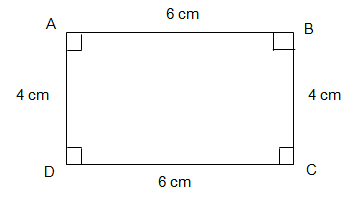a) Square
b)  Rectangle

Q.7) If the two angles of the Triangle are ∠A = 40˚ , ∠C = 65˚, find ∠B ?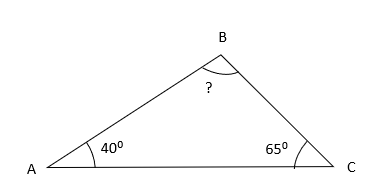a) ∠B = 65˚
b) ∠B = 85˚
c) ∠B = 75˚

Q.8) In the given figure , find x˚ and y˚ ?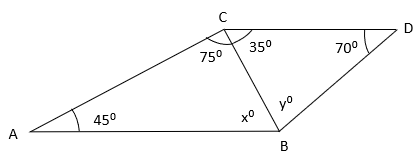a) x˚ = 50˚ , y˚ = 85˚
b) x˚ = 70˚ , y˚ = 65˚
c) x˚ = 60˚ , y˚ = 75˚

### Polygons Worksheet Grade 5 Explanations

Q.1) Explanation – Polygons Worksheet Grade 5

In the given figure
Sides of the Triangle are
AB    =    7 cm
BC    =    5 cm
AC    =    6 cm
We know that,
If all the sides of a Triangle are unequal, it is called a Scalene Triangle.
In the given figure all the sides of the Triangle are unequal.
Therefore, the given Triangle is a Scalene Triangle.

Correct Answer – c) Scalene Triangle

Q.2) Explanation – Polygons Worksheet Grade 5

In the given figure
The Sides of the triangle are
AB    =    4 cm
BC    =    4 cm
AC    =    6 cm
We know that,
If two of the sides of a Triangle are equal, it is called an Isosceles Triangle.
In the given figure, sides AB and BC of the Triangle are equal.
Therefore, the given Triangle is an Isosceles Triangle.

Correct Answer – b) Isosceles Triangle

Q.3) Explanation – Polygons Worksheet Grade 5

In the given figure
Sides of triangle are
AB    =    5 cm
BC    =    5 cm
AC    =    5 cm
We know that,
If all the sides of a Triangle are equal, it is called an Equilateral Triangle.
In the given Triangle all the sides i.e. AB, BC and AC are equal.
Therefore, the given Triangle is an Equilateral Triangle.

Correct Answer – a) Equilateral Triangle

Q.4) Explanation – Polygons Worksheet Grade 5

In the given figure
The three angles of the Triangle are, 30˚, 60˚ and 90˚
We know that,
If one angle of a triangle is equal to 90˚, it is called a Right Angled Triangle.
In the given figure one of the angles in the Triangles is equal to 90˚
Therefore, the given Triangle is a Right Angled Triangle.

Correct Answer – c) Right Angled Triangle

Q.5) Explanation – Polygons Worksheet Grade 5

In the given figure
The three angles of the Triangle are 30˚, 30˚ and 120˚
We know that,
If one angle of a triangle is more than 90˚ (known as Obtuse angle), It is called an Obtuse Angled Triangle.
In the given figure one of the angles is 120˚, which is more than 90˚
Therefore, the given Triangle is an obtuse angled Triangle.

Correct Answer – a) Obtuse Angled Triangle

Q.6) Explanation – Polygons Worksheet Grade 5

Square – Square is a 2D shape in which all the sides are equal and each angle is of 90˚.
Rectangle – Rectangle is 2D shape is which Opposite sides are equal and each angle is 90˚.
In the given figure,
AB = CD = 6 cm and AD = BC = 4 cm and each angle is Right angle
Therefore, the given figure is a Rectangle (Since all the sides are not equal, it is not a square).

Q.7) Explanation – Polygons Worksheet Grade 5

In the given figure,∠A = 40˚
∠C = 65˚
∠B = ?
We know that,
Sum of all the angles of Triangle = 180˚
Therefore,
∠A + ∠B + ∠C = 180˚
40˚ + 65˚ + ∠B = 180˚
105˚ + ∠B = 180˚
∠B = 180˚ – 105˚
∠B = 75˚

Correct Answer – c) ∠B = 75˚

Q.8) Explanation – Polygons Worksheet Grade 5

In the given figure,In Δ ABC
∠A = 45˚
∠ABC = x˚
∠ACB = 75˚
We know that,
Sum of all the three angles of Traingle = 180˚
∠A + ∠ABC + ∠ACB = 180˚
45˚ + x˚ + 75˚ = 180˚
120˚ + x˚ = 180˚
x˚ = 180˚ – 120˚
x˚ = 60˚
Similarly,
In Δ BCD
∠BCD = 35˚
∠CBD = y˚
∠D = 70˚
∠BCD + ∠CBD + ∠D = 180˚
35˚ + y˚ + 70˚ = 180˚
105˚ + y˚ = 180˚
y˚ = 180˚ – 105˚
y˚ = 75˚

Correct Answer – c) x˚ = 60˚ , y˚ = 75˚